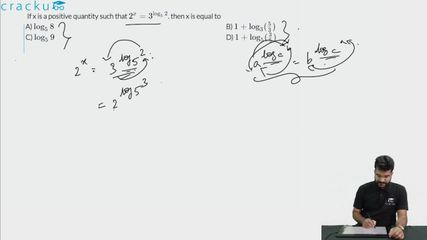Question 67

# If x is a positive quantity such that $$2^{x}=3^{\log_{5}{2}}$$. then x is equal to

Solution

Givne that: $$2^{x}=3^{\log_{5}{2}}$$

$$\Rightarrow$$ $$2^{x}=2^{\log_{5}{3}}$$

$$\Rightarrow$$ $$x=\log_{5}{3}$$

$$\Rightarrow$$ $$x=\log_{5}{\dfrac{3*5}{5}}$$

$$\Rightarrow$$ $$x=\log_{5}{5}+\log_{5}{\dfrac{3}{5}}$$

$$\Rightarrow$$ $$x=1+\log_{5}{\dfrac{3}{5}}$$. Hence, option D is the correct answer.

### View Video Solution• All Quant CAT Formulas and shortcuts PDF
• 30+ CAT previous papers with solutions PDF

##### Formi Nagar

1 year, 2 months ago

Its a different question

##### Diptimayee Sethi

1 week, 2 days ago

The sequence is just different

1 year ago

Right